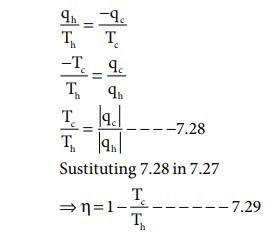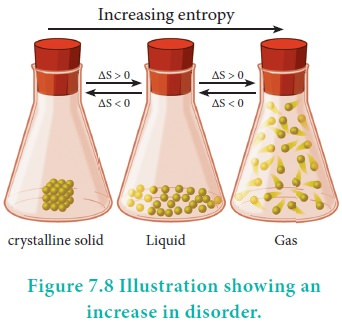Home | | Chemistry 11th std | Second Law of thermodynamics

# Second Law of thermodynamics

The second law of thermodynamics introduces another state function called entropy.

Second Law of thermodynamics:

## Need for the second law of thermodynamics:

We know from the first law of thermodynamics, the energy of the universe is conserved. Let us consider the following processes:

1. A glass of hot water over time loses heat energy to the surrounding and becomes cold.

2. When you mix hydrochloric acid with sodium hydroxide, it forms sodium chloride and water with evolution of heat.

In both these processes, the total energy is conserved and are consistent with the first law of thermodynamics. However, the reverse process i.e. cold water becoming hot water by absorbing heat from surrounding on its own does not occur spontaneously even though the energy change involved in this process is also consistent with the first law. However, if the heat energy is supplied to cold water, then it will become hot. i.e. the change that does not occur spontaneously and an be driven by supplying energy.

Similarly, a solution of sodium chloride does not absorb heat energy on its own, to form hydrochloric acid and sodium hydroxide. But, this process can not be driven even by supplying energy. From these kinds of our natural experiences, we have come to know that certain processes are spontaneous while the others are not, and some processes have a preferred direction. In order to explain the feasibility of a process, we need the second law of thermodynamics.

## Various statements of the second law of thermodynamics

### Entropy

The second law of thermodynamics introduces another state function called entropy. Entropy is a measure of the molecular disorder (randomness) of a system. But thermodynamic definition of entropy is concerned with the change in entropy that occurs as a result of a process.

It is defined as, dS = dqrev / T

### Entropy statement:

The second law of thermodynamics can be expressed in terms of entropy. i.e ŌĆ£the entropy of an isolated system increases during a spontaneous processŌĆØ.

For an irreversible process such as spontaneous expansion of a gas,

ŌłåStotal > 0

ŌłåStotal > ŌłåSsystem + ŌłåSsurrounding

i.e. ŌłåSuniverse > ŌłåSsystem + ŌłåSsurroundingFor a reversible process such as melting of ice,

ŌłåSsystem = - ŌłåSsurrounding

ŌłåSuniverse = 0

### Kelvin-Planck statement:

It is impossible to construct a machine that absorbs heat from a hot source and converts it completely into work by a cyclic process without transferring a part of heat to a cold sink. The second law of thermodynamics explains why even an ideal, frictionless engine cannot convert 100% of its input heat into work. Carnot on his analysis of heat engines, found that the maximum efficiency of a heat engine which operates reversibly, depends only on the two temperatures between which it is operated.

Efficiency = work performed / heat absorbedqh - heat absorbed from the hot reservoir

qc - heat transferred to cold reservoir

For a reversible cyclic process

╬öSuniverse = ╬öSsystem + ╬öSsurroundings = 0

╬öSsystem = - ╬öSsurroundingsTh >> Tc

Hence, ╬Ę < 1

### efficiency in percentage can be expressed as### Clausius statement:

It is impossible to transfer heat from a cold reservoir to a hot reservoir without doing some work.

### Problem: 7.10

If an automobile engine burns petrol at a temperature of 816o C and if the surrounding temperature is 21o C, calculate its maximum possible efficiency.

### Solution:Here

Th = 816+273= 1089 K;

Tc= 21+273= 294K

%Efficiency=( 1089-294 / 1089) x100

%Efficiency=73%

### Unit of entropy:

The entropy (S) is equal to heat energy exchanged (q) divided by the temperature (T) at which the exchange takes place. Therefore, The SI unit of entropy is JKŌłÆ1.

### Spontaneity and Randomness

Careful examination shows that in each of the processes viz., melting of ice and evaporation of water, there is an increase in randomness or disorder of the system. The water molecules in ice are arranged in a highly organised crystal pattern which permits little movement. As the ice melts, the water molecules become disorganised and can move more freely. The movement of molecules becomes freer in the liquid phase and even more free in the vapour phase. In other words, we can say that the randomness of the water molecules increases, as ice melts into water or water evaporates. Both are spontaneous processes which result in a increase in randomness (entropy).### Standard Entropy Change(╬öS0):

It is possible to calculate the actual entropy of a substance at any temperature above 0 K. The absolute entropy of a substance at 298 K and one bar pressure is called the standard entropy So. The third law of thermodynamics states, according to Nernst, that the absolute entropy of elements is zero only at 0 K in a perfect crystal, and standard entropies of all substances at any temperature above 0 K always have positive values. Once we know the entropies of different substances, we can calculate the standard entropy change ( Sr0 ) for chemical reactions.

S0r = Ōłæ S0products ŌłÆ Ōłæ Sreac0 tan ts ------- (7.30)

### Standard Entropy of Formation:

Standard entropy of formation is defined as ŌĆ£the entropy of formation of 1 mole of a compound from the elements under standard conditionsŌĆØ. It is denoted as ŌłåS0f . We can calculate the value of entropy of a given compound from the values of S0 of elements.

### Problem: 7.6

Calculate the standard entropy change for the following reaction( ŌłåS0f ), given the standard entropies of CO2(g), C(s),O2(g) as 213.6 , 5.740 and 205 JKŌłÆ1 respectively.

C(g) + O2(g) ŌåÆCO2(g)

S0r = Ōłæ S0products ŌłÆ Ōłæ Sreac0 tan ts

S0r = {S0CO 2 } ŌłÆ {SC0 + S0O2 }

S0r = 213.6 ŌłÆ [5.74 + 205]

S0r = 213.6 ŌłÆ[210.74]

S0r = 2.86 JKŌłÆ1

### Entropy change accompanying change of phase

When there is a change of state from solid to liquid (melting), liquid to vapour (evaporation) or solid to vapour (sublimation) there is a change in entropy. This change may be carried out at constant temperature reversibly as two phases are in equilibrium during the change.### Entropy of fusion:

The heat absorbed, when one mole of a solid melts at its melting point reversibly, is called molar heat of fusion. The entropy change for this process is given bywhere ╬öHfusion is Molar heat of fusion. Tf is the melting point.

### Entropy of vapourisation:

The heat absorbed, when one mole of liquid is boiled at its boiling point reversibly, is called molar heat of vapourisation. The entropy change is given bywhere ╬öHv is Molar heat of vaporisation.

Tb is the boiling point.

### Entropy of transition:

The heat change, when one mole of a solid changes reversibly from one allotropic form to another at its transition temperature is called enthalpy of transition. The entropy change is givenwhere ╬öHt is the molar heat of transition, Tt is the transition temperature.

Problem: 7.7

Calculate the entropy change during the melting of one mole of ice into water at 00 C and 1 atm pressure. Enthalpy of fusion of ice is 6008 J mol-1

Given:

ŌłåHfusion = 6008 JmolŌłÆ1

Tf = 0 0

C = 273 K

H 2O(S) --273 KŌåÆ H 2O ( l)S fusion = 22 .007 J K ŌłÆ1 moleŌłÆ1

Tags : Need, Various statements, Solved Example Problems , 11th Chemistry : UNIT 7 : Thermodynamics
Study Material, Lecturing Notes, Assignment, Reference, Wiki description explanation, brief detail
11th Chemistry : UNIT 7 : Thermodynamics : Second Law of thermodynamics | Need, Various statements, Solved Example Problems